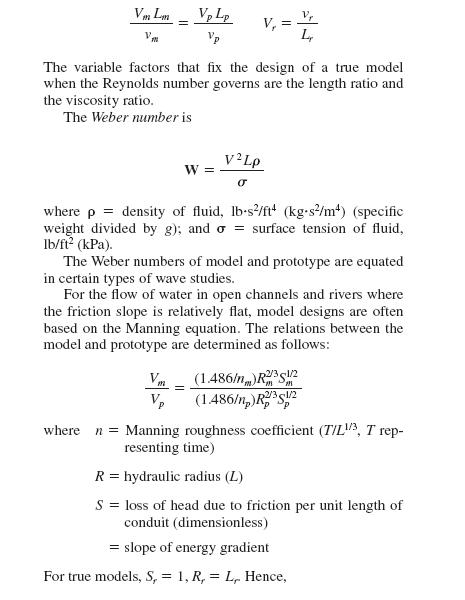Awarded as the best online publication by CIDC

# Similitude for physical models

A physical model is a system whose operation can be used to predict the characteristics of a similar system, or prototype, usually more complex or built to a much larger scale.”A model can be either smaller or bigger than the real construction.It is believed that model is always smaller but that is not true always for example if we want to make a very small computer chip then to illustrate its function properly the model is made bigger as compared to the original chip.
Ratios of the forces of gravity, viscosity, and surface tension to the force of inertia are designated, Froude number, Reynolds number, and Weber number, respectively. Equating the Froude number of the model and the Froude number of the prototype ensures that the gravitational and inertial forces are in the same proportion. Similarly, equating the Reynolds numbers of the model and prototype ensures that the viscous and inertial forces are in the same proportion. Equating the Weber numbers ensures proportionality of surface tension and inertial forces.

F=V/(Lg)1/2

where

F= Froude number (dimensionless)

V= velocity of fluid, ft/s (m/s)

L= linear dimension (characteristic, such as depth or diameter), ft (m)

g= acceleration due to gravity, 32.2 ft/s2 (9.81 m/s2)

For hydraulic structures, such as spillways and weirs, where there is a rapidly changing water-surface profile, the two predominant forces are inertia and gravity. Therefore, the
Froude numbers of the model and prototype are equated:

Fm=Fp

Vm/(Lmg)1/2=Vp/(Lpg)1/2

Viscous forces are usually predominant when flow occurs in a closed system, such as pipe flow where there is no free surface. The following relations are obtained by equating Reynolds numbers of the model and prototype: